# Find the radius of a closed right circular cylinder of volume

Question:

Find the radius of a closed right circular cylinder of volume $100 \mathrm{~cm}^{3}$ which has the minimum total surface area.

Solution: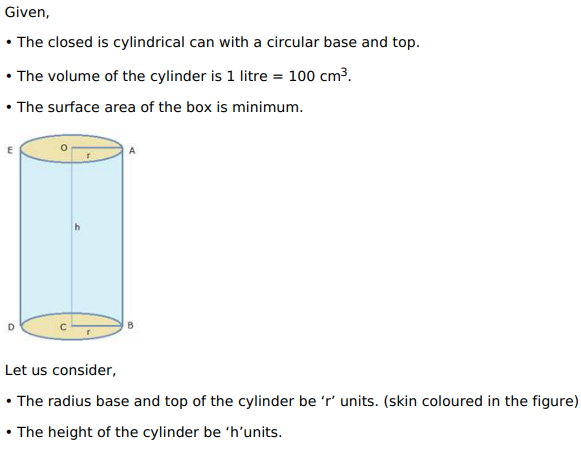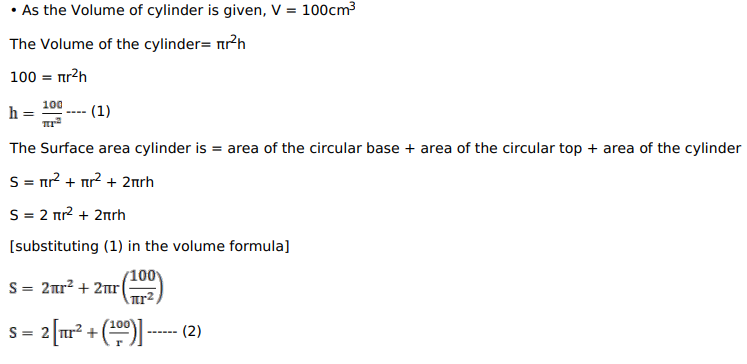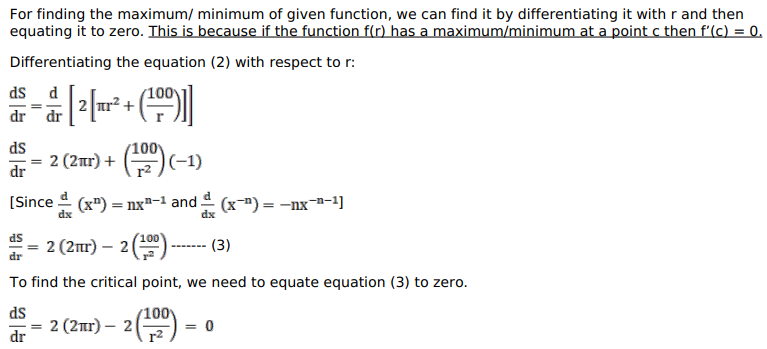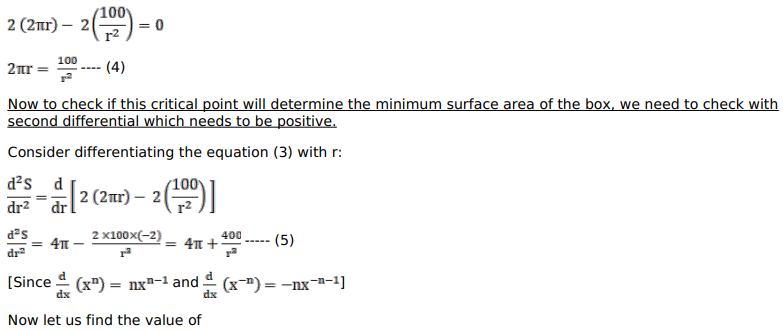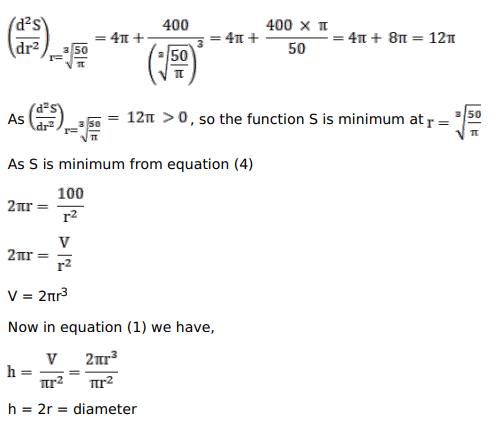Therefore when the total surface area of a cone is minimum, then height of the cone is equal to twice the radius or equal to its diameter.